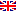Scheda programma d'esame
TEORIA GEOMETRICA DELLA MISURA
GIOVANNI ALBERTI
CdSMATEMATICA
Codice225AA
CFU6
PeriodoSecondo semestre
LinguaItaliano

ModuliSettore/iTipoOreDocente/i
TEORIA GEOMETRICA DELLA MISURA/aMAT/05LEZIONI42
 GIOVANNI ALBERTI unimapEsporta in pdf
Learning outcomes
Knowledge

At the end of this course the student should know the foundations of Geometric Measure Theory (Hausdorff measures, characterization of dimension, rectifiable sets) and some of its applications (finite perimeter sets).

Assessment criteria of knowledge

Final oral examination.

Skills

At the end of the course the student should be able to understand at least the introduction of a publication on the subject. He should also be able to complete a proof starting from a sketch, and fill-in the technical details omitted in the lectures.

Prerequisites

The standard analysis and geometry courses required for the first degree in Mathematics in Pisa, and in particular the basic theory of Measure and Integration according to Lebesgue. An advanced course on the foundations of  functional analysis, including the basic theory of Sobolev spaces.

Teaching methods

The course will consist of frontal lectures given in English.

Syllabus
• Basics of measure theory: covering theorems, Radon-Nikodým theorem, external measures and Caratheodory construction.
• Hausdorff measure and dimension according, and their fundamental properties. Structure of measures with finite and positive d-dimensional densities. Hutchinson's self-similar fractals.
• Lipschitz functions. Rectifiable sets. Approximate tangent to a rectifiable set. Rectifiability  criteria. Area formula.
• Functions of bounded variation in several variables (BV). Finite perimeter sets, definition, basic properties, compactness. Essential boundary and structure of the derivative of finite perimeter sets. Basic regularity for minimal perimeter sets.
• Applications: existence theorems for the Plateau problem (in codimension one), for capillarity problems, for minimal clusters.
• Fine properties of BV functions: approximation and approximate continuity. SBV functions and Ambrosio compactness theorem. Existence of minimizers for the Mumford-Shah functional.
Bibliography
• K. Falconer: The geometry of fractal sets. Cambridge University Press, 1985.
• P. Mattila: Geometry of sets and measures in Euclidean spaces. Cambridge University Press, 1995.
• L. Ambrosio, N. Fusco, D. Pallara: Functions of bounded variation and free discontinuity problems. Oxford University Press, 2000.
• S.G. Krantz, H.R. Parks: Geometric Integration Theory. Cornerstones. Birkhäuser, Boston 2008.
Assessment methods

The final exam consists of two parts: a seminar prepared by the student on a topic proposed by the teacher, and a standard ora examintation.